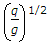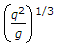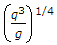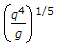# Mechanical Engineering - Hydraulics and Fluid Mechanics

### Exercise :: Hydraulics and Fluid Mechanics - Section 4

16.

The intensity of pressure at any point, in a liquid, is

 A. directly proportional to the area of the vessel containing liquid B. directly proportional to the depth of liquid from the surface C. directly proportional to the length of the vessel containing liquid D. inversely proportional to the depth of liquid from the surface

Answer: Option B

Explanation:

No answer description available for this question. Let us discuss.

17.

If the depth of water in an open channel is greater than the critical depth, the flow is called

 A. critical flow B. turbulent flow C. tranquil flow D. torrential flow

Answer: Option C

Explanation:

No answer description available for this question. Let us discuss.

18.

A submerged body is said to be in a stable equilibrium, if its centre of gravity __________ the centre of buoyancy.

 A. coincides with B. lies below C. lies above

Answer: Option B

Explanation:

No answer description available for this question. Let us discuss.

19.

The critical depth for a channel is given by (where q = Unit discharge (discharge per unit width) through the channel)

 A.B.C.D.Answer: Option B

Explanation:

No answer description available for this question. Let us discuss.

20.

The liquid used in manometers should have

 A. low density B. high density C. low surface tension D. high surface tension

Answer: Option D

Explanation:

No answer description available for this question. Let us discuss.

#### Current Affairs 2021

Interview Questions and Answers